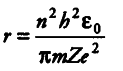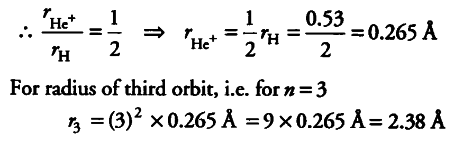# N Bohr’s model of H-atom. the radius of the first electron orbit is 0.53 A

In Bohr’s model of H-atom. the radius of the first electron orbit is 0.53 A. What will be the radius of the third orbit and the first orbit of singly ionised helium atom?

Radius of nth Bohr’s orbit =Again , r ∝ 1/z
{ r }_{ He+ } / Rhe =
{ Z }_{ H+ } / Zhe+
For hydrogen , Z=1 and for helium , Z=2